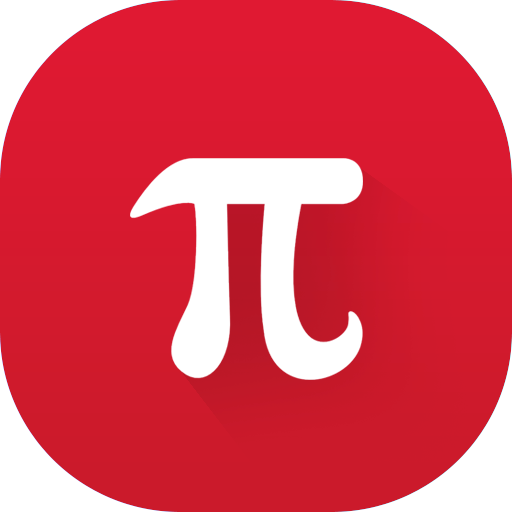## Maths - Mathematics & Equation Solver

### by Best app makerz

Free
425
Categories Tags
###### Description

Maths - Mathematics & Equation Solver is a math problem solver with step by step description and graph view for functions. Totally free & works offline too!!

Maths - Mathematics & Equation Solver covers:

. Basic Math
. Algebra
. Trigonometry
. Precalculs
. Calculus
. Statistics
. Finite Math
. Linear Algebra
. Chemistry
. Graphing
. and more..

Maths - Mathematics & Equation Solver is a scientific graphing calculator integrated with algebra and is an indispensable mathematical tool for students from high school to those in college or graduate school or even university!

870

1913
• AOTD

2187